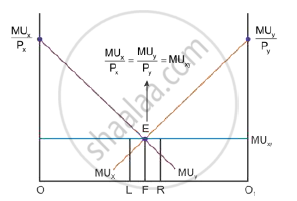# A Consumer Consumes Only Two Goods. Explain Consumer'S Equilibrium with the Help of Utility Analysis. - Economics

A consumer consumes only two goods. Explain consumer's equilibrium with the help of utility analysis.

A consumer consumes only two goods X and Y. Explain the conditions of consumer’s equilibrium using Marginal Utility Analysis.

A consumer consumes only two goods. Explain the conditions of consumers’ equilibrium using utility analysis.

#### Solution

Conditions of consumer’s equilibrium using marginal utility analysis:

When a consumer buys both Goods X and Y, the consumer’s equilibrium condition is expressed through the equation:

(MU_x)/P_x=(MU_y)/P_y=(MU_m)/P_n=MU_m

Consider the following numerical example to understand the consumer’s equilibrium using marginal utility. A consumer Marginal Utility of Money (MUm) is 16 utils and two Goods X and Y whose prices are Rs 1 (Px) and Rs 1 (Py) per unit, respectively. Consider the following schedule to analyse the marginal utility of good x (MUx) and good y (MUy).

 Units of x MU x (Utils) MU y3 (Utils) 1 28 32 2 24 29 3 21 27 4 20 23 5 16 20 6 13 18 7 9 17 8 5 16 9 3 12 10 1 9

Based on the given schedule, the consumer is in equilibrium at the consumption of 5 units of commodity x and 8 units of commodity y. At such a consumption combination, the

marginal utility of a rupee spent on the commodity x((MU_x)/P_x) is equal to the marginal utility of a rupee spent on the commodity y((MU_y)/P_y)and also equal to the marginal utility of money (MUm).

Marginal utility of a rupee spent on commodity x = marginal utility of a rupee spent on commodity y = Marginal utility of money

(MU_x)/P_x=(MU_y)/P_y=MU_m

(MU_x)/P_x=(MU_y)/P_y=16/1=16=MU_mIn the diagram, OO1 is the total income of a consumer. MUx and MUy are the marginal utility curves of commodity x and commodity y, respectively.

The consumer does not attain equilibrium at Point L because the point at L is

(MU_x)/P_x>(MU_y)/P_y

The consumer does not attain equilibrium at Point R because the point at R is

(MU_x)/P_x<(MU_y)/P_y

So, when OF amount of income is spent on commodity x and FO1 amount is spent on commodity y, the consumer is in equilibrium at Point E. Hence, at this point

(MU_x)/P_x=(MU_y)/P_y=MU_m

Concept: Conditions of Consumer's Equilibrium Using Marginal Utility Analysis
Is there an error in this question or solution?
2013-2014 (March) Delhi Set 1
Share